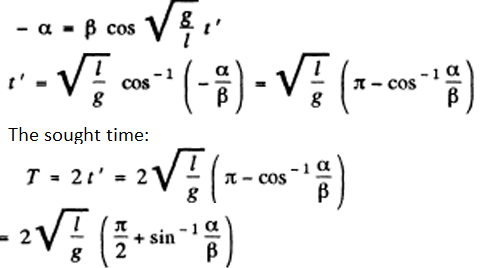Checkout JEE MAINS 2022 Question Paper Analysis : Checkout JEE MAINS 2022 Question Paper Analysis :

# I.E. Irodov Solutions on Mechanical Oscillations

I.E. Irodov Solutions on Chapter 4.1 Mechanical Oscillation is available on this page. I.E. Irodov Solutions on Mechanical Oscillation is a very important chapter and consists of questions which are frequent in competitive examinations. A mechanical oscillation is a periodic conversion of energy from potential energy to kinetic energy to potential energy etc. This chapter is based on different concepts such as electromagnetic wave propagates, magnetic component of the wave, oscillation period, Maxwell's equations, current densities and many more. In order to help students master the concepts discussed in chapter 4.1 of I.E. Irodov book and develop better problems skills, we are offering free solutions on this page.

### I.E. Irodov Solutions on Mechanical Oscillations

1: A point oscillates along the x axis according to the law x = a cos (ωt - 𝜋/4). Draw the approximate plots

(a) of displacement x, velocity projection vx, and acceleration projection wx as functions of time t;

(b) velocity projection vx and acceleration projection wx as functions of the coordinate x.

Solution:

1.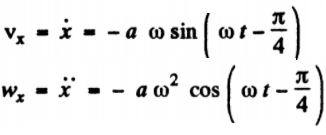…(1)

(b) From equation (1)

vx = -a ω cos (ωt - 𝜋/4) so, vx2 = -a2 ω2 sin2 (ωt - 𝜋/4) ….(2)

But from the law x = a cos (ωt - π/4), so, x2 = a2 cos2 (ωt - π/4)

or, cos (ωt - π/4) = x2/a2 or sin2 (ωt - π /4) = 1- x2/a2 …..(3)

Using (3) in (2),

vx2 = a2 ω2(1 - x2/a2) or vx2 = ω2(a2 - x2) ….(4)

Again, from Equation (4), wx = -aω2cos (ωt - π/4) = -ω2x

2: A point moves along the x axis according to the law x = a sin2(ωt-π/4). Find:

(a) the amplitude and period of oscillations; draw the plot x (t);

(b) the velocity projection vx as a function of the coordinate x.

Solution:

1. (a) From the motion law of the particle

x = a sin2(ωt-π/4) = (a/2)[1 – cos(2ωt - π/2)]

x – a/2 = (-a/2) cos(2ωt - π/2) = (-a/2) sin(2ωt) = (a/2) sin(2ωt + π)

that is, x – a/2 = (a/2) sin(2ωt + π) ….(1)

Now comparing this equation with the general equation of harmonic oscillations: X - A sin(ωot + a) and Amplitude, A = a/2 and angular frequency, ω0 - 2ω.

Thus, the period of one full oscillation, T = π/ω

(b) Differentiating Equation (1) w.r.t. time

vx = aω cos(2ωt + π) or vx2 = a2ω2 cos2 (2ωt + π) = a2ω2 [1-sin2 (2ωt + π)] …(2)

From equation (1), (x – a/2)2 = (a2/4) sin2 (2ωt + π)

Or 4x2/a2 + 1 - 4x/a = sin2 (2ωt + π) or 1- sin2 (2ωt + π) = (4x/a)(1 – x/a) ….(3)

From (2) and (3), vx = 4ω2x(a-x)

3: A particle performs harmonic oscillations along the x axis about the equilibrium position x = 0. The oscillation frequency is ω = 4.00 s-1. At a certain moment of time the particle has a coordinate xo = 25.0 cm and its velocity is equal to vx0 = 100 cm/s. Find the coordinate x and the velocity vx of the particle t = 2.40s after that moment.

Solution:

1. h = a cos (ωt + α)

So, vx = - a ω sin (ωt + α)

Let us assume that at t = 0 , x = h0 and vx = vxo.

Thus from Equations (1) and (2) for t = 0, h0 = a cos α, and vxo = - a ω sin α

Therefore, tan α = - vxo/ωxo and

$$a = \sqrt{x_o + (\frac{v_{xo}}{\omega})^2 }$$
= 35.35 cm

Under our assumption equations (1) and (2) give the sought x and vx, if

t = 2.40 s and

$$a = \sqrt{x_o + (\frac{v_{xo}}{\omega})^2 }$$

and α = tan-1(-vx/ ωxo) = - π/4

Substituting the values, we get

x = -29 cm and vx = -81 cm/s.

4: Find the angular frequency and the amplitude of harmonic oscillations of a particle if at distances x1 and x2 from the equilibrium position its velocity equals v1 a nd v2 respectively.

Solution:

1. we know,

vx2 = ω2 (a2 - x2)

v12 = ω2 (a2 – x12) and v22 = ω2 (a2 – x22)

Solving above equations, we have

$$\omega = \sqrt{\frac{v_1^2- v_2^2}{ x_2^2- x_1^2}}$$
and

$$a = \sqrt{\frac{v_1^2 x_2^2- v_2^2 x_1^2}{ v_1^2- v_2^2}}$$

5: A point performs harmonic oscillations along a straight line with a period T = 0.60 s and an amplitude a = 10.0 cm. Find the mean velocity of the point averaged over the time interval during which it travels a distance a/2, starting from (a) the extreme position; (b) the equilibrium position.

Solution:

1. (a) When a particle starts from an extreme position, it is useful to write the motion law as

x = a cos ωt .......(1)

If tx be the time to cover the distance a/2 then from (1)

( x is the displacement from the equilibrium position)

a-a/2 = a/2 = a cos ωt1 or cos ωt1 = (1/2) cos(𝜋/3) [as t1 < T/4]

Therefore, t1 = 𝜋/3ω = 𝜋/3(2 𝜋/T) = T/6

As, x = a cos ωt, so vx = -a ω sin ωt

Therefore, v= |vx| = vx = a ω sin ωt for t ≤ t1 = T/6

Mean velocity is, <v>

$$\frac{\int vdt}{\int dt} = \int_0^{T/6}(2 \pi/T) sin \omega t dt$$
/T/6 = 3a/T = 0.5 m/s

(b) Let us write the motion law in the form: x = a ω sin ωt …(2)

If t2 be the time to cover the distance a/2, then from Equation (2)

(a/2) = a sin (2 𝜋/T) t2 or sin (2 𝜋/T) t2 = (1/2) sin 𝜋/6 (as t2 < T/4)

(2 𝜋/T)t2 = 𝜋/6 or t2 = T/12

On differentiating (2) with respect to time,

Vx =a ωcos ωt = a 2 𝜋/T cos(2 𝜋/T)t

Or, v = |vx| = a (2 𝜋/T) cos(2 𝜋/T)t for t ≤ t2 = T/12

Mean velocity is, <v>

$$\frac{\int vdt}{\int dt} = 1/(T/12) \int_0^{T/12}(a2 \pi/T) cos (2\pi/T) t dt$$
= 6a/T = 1 m/s

6: At the moment t = 0 a point starts oscillating along the x axis according to the law x = a sin ωt. Find:

(a) the mean value of its velocity vector projection (vs).

(b) the modulus of the mean velocity vector |(v)| ;

(c) the mean value of the velocity modulus (v) averaged over 3/8 of the period after the start.

Solution:

1. As x = a sin ωt, so vx = aω cos ωt

Thus,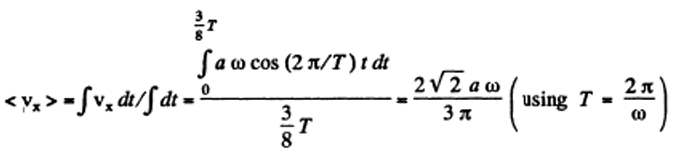(b)

$$\vec{v} = v_xi$$
, so
$$|<\vec{v}>| = |<v_x>|$$
|

Using part (a),

$$|<\vec{v}>| = |\frac{2\sqrt{2}a\omega}{3\pi}| = \frac{2\sqrt{2}a\omega}{3\pi}$$

(c) vx = aω cos ωt

So,

v = |vx| = aω cos ωt for t ≤ T/4

v = |vx| = -aω cos ωt for T/4 ≤ t ≤ (3/8)T

hence,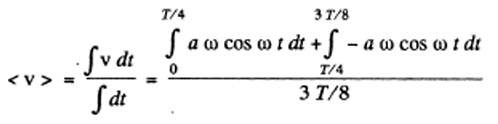Using ω = 2 ω/T, and on evaluating the integral we get <v> = [2(4- √2)aω]/3π

7: A particle moves along the x-axis according to the law x = a cos ωt. Find the distance that the particle covers during the time interval from t = 0 to t.

Solution:

1. From the motion law, x = a cos ωt it is obvious that the time taken to cover the distance equal to the amplitude (a), starting from extreme position equals T/4.

Now,

t = n(T/4) + to (where to <T/4 and n = 0,1,2,3,…)

As the particle moves according to the law, x = a cos ωt, so at n = 1,3,5 ... or for odd n values it passes through the mean positon and for even numbers of n it comes to an extreme position (if t0 = 0).

Case (1) when n is an odd number: In this case, from the equation, x = x a sin ωt, if the t is counted from n T/4 and the distance covered in the time interval to be comes

s1= asin ωt0 = asin ω(t – nT/4) = asin (ωt - n π/2)

Distance covered for odd n is

s= na + s1 = na + a sin(ωt - n π/2) = a[n + sin(ωt - n π/2)]

Case (2), when n is even, In this case from the equation x = a cos ωt, the distance covered ( s2 ) in the interval t0, is given by

a-s2= a cos(ωt0) = a cos(ωt - n T/4) = a cos(ωt - n π /2)

s2=a[1 - cos(ωt - n π /2)]

Distance covered for n is even :

s= na + s2 = na + a[1 - cos(ωt - n π/2) = a[n +1 - cos(ωt - n π/2)]

In general: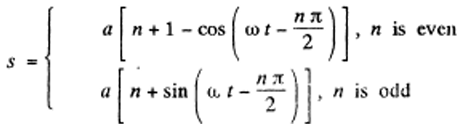8: At the moment t = 0 a particle starts moving along the x-axis so that its velocity projection varies as vx = 35 cos πt cm/s, where t is expressed in seconds. Find the distance that this particle covers during t = 2.80 s after the start.

Solution:

1. The motion law is of the from, x = a shoot, and vx = ω a cos ωt.

Comparing vx - ω a cos ωt with vx - 35 cos πt , we have

ω =π, a = 35/π thus T = 2π/ω = 2 and T/4 = 0.5 s

Now we can write , t = 2.8 s = 5 x T/4 + 0.3

(where T/4 = 0.5 s)

As n = 5 is odd, like (4 = 7), we have to basically find the distance covered by the particle starting from the extreme position in the time interval 0 = 3 s.

Therefore, x = a cos ωt = (35/ π) cos π(0.3)

35/ π – s1 = (35/ π) cos π(0.3) or s1 = (35/ π) [1 - cos π(0.3)]

The sought distance is,

s= 5 x (35/ π) + (35/ π) [1 - cos π(0.3)]

= (35/ π) [6 - cos π(0.3)]

= (35/22) x 7 [6 - cos 54o]

= 60 cm (approx.)

9: A particle performs harmonic oscillations along the x-axis according to the law x = a cos ωt. Assuming the probability P of the particle to fall within an interval from -a to +a to be equal to unity, find how the probability density dP/dx depends on x. Here dP denotes the probability of the particle falling within an interval from x to x dx. Plot dP/dx as a function of x.

Solution:

1. As the motion is periodic the particle repeatedly passes through any given region in the range - a ≤ x ≤ a.

The probability that it lies in the range (x, x + dx) is defined as the fraction

∆t/t (as t-> infinity)

where Δt is the time that the particle lies in the range (x, x + dx) out of the total time t. Because of periodicity this is

dP = (dP/dx) dx = dt/T = 2dx/vT

where the factor 2 is needed to take account of the fact that the particle is in the range ( x , x + d x ) during both up and down phases of its motion. Now in a harmonic oscillator.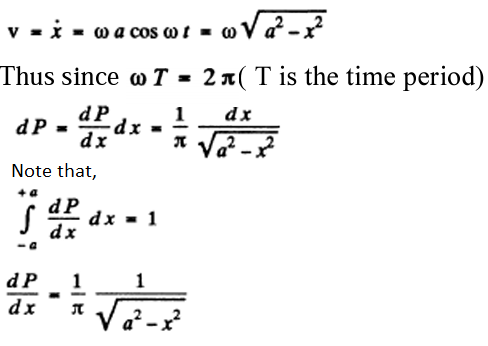10: Using graphical means, find an amplitude a of oscillations resulting from the superposition of the following oscillations of the same direction:

(a) x1 =3 .0 cos (ωt -1- π/3), x2 = 8 .0 sin (ωt + π/6);

(b) x1 = 3.0 cos ωt, x2 = 5.0 cos (ωt+ π/4), x3 =6 .0 sin ωt.

Solution:

1. Choose an axis (X - axis) and an origin. Draw a vector of magnitude 3 inclined at an angle π/3 with the X-axis. Draw another vector of magnitude 8 inclined at an angle - π/3 (Since sin (ωt+ π/ 6 ) >> cos (ωt- π/ 3 )) with the X - axis. The magnitude of the resultant of both these vectors (drawn from the origin) obtained using parallelogram law is the resultant, amplitude.So, R2 = 32 + 82 + 2.3.8 cos (2 π/3)

Or R = 7 units

(b) One can follow the same graphical method here but the result can be obtained more quickly by breaking into sines and cosines and adding:

Resultant , x = (3 + 5/ √2)cosωt + (6 - 5/ √2)sin ωt = A cos(ωt+α)

Then, A2 = (3 + 5/ √2)2+ (6 - 5/ √2)2 = 9 + 25 + (30-60)/√2 + 36

or A = 7 units

Note: In using graphical method convert all oscillations to either sines or cosines but do not use both.

11: A point participates simultaneously in two harmonic oscillations of the same direction: x1 = a cos ωt and x2 = a cos 2ωt. Find the maximum velocity of the point.

Solution:

1. The net displacement,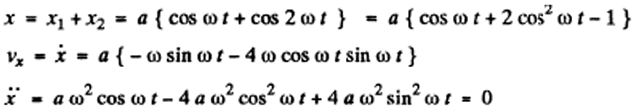(for dot x to be maximum)

Solving for acceptable value : cos ωt = 0.644

Thus, sin ωt = 0.765 and

vmax = |vxmax| = +aω[0.765 + 4 x 0.765 x 0.644] = +2.73 aω.

12: The superposition of two harmonic oscillations of the same direction results in the oscillation of a point according to the law x = a cos 2.1t cos 50.0t, where t is expressed in seconds. Find the angular frequencies of the constituent oscillations and the period with which they beat.

Solution:

1. a cos 2.1 t cos 50.0 t = (a/2)[cos 52.1 t + cos 47.9 t]

The angular frequencies of constituent oscillations are 52.1 s-1 and 47.9 s-1

To get the beat period note that the variable amplitude a cos 2.1t becomes maximum (positive or negative), when

2.1 t = nπ,

The interval between two maxima is, π/2.1 = 1.5 s nearly.

13: A point A oscillates according to a certain harmonic law in the reference frame K' which in its turn performs harmonic oscillations relative to the reference frame K. Both oscillations occur along the same direction. When the K' frame oscillates at the frequency 20 or 24 Hz, the beat frequency of the point A in the K frame turns 167 out to be equal to v. At what frequency of oscillation of the frame K' will the beat frequency of the point A become equal to 2v?

Solution:

1. If the frequency of A with respect to K' is v0 and K ' oscillates with frequency

$$\bar{v}$$
with respect to K, the beat frequency of the point A in the K-frame will be v when
$$\bar{v} = v_0 \pm v$$
.

In the present case,

$$\bar{v}$$
= 20 or 24.

This means v0 - 22 and v - 2

Thus, beats of 2v = 4 will be heard when

$$\bar{v}$$
= 26 or 18.

14: A point moves in the plane xy according to the law x = a sin ωt, y = b cos ωt, where a, b, and ω are positive constants. Find: (a) the trajectory equation y (x) of the point and the direction of its motion along this trajectory; (b) the acceleration ω of the point as a function of its radius vector r relative to the origin of coordinates.

Solution:

1. (a) From the Equation : x = a sin ωt

Sin2ω t = x2/a2 or cos2ω t = 1- x2/a2 ……(1)

And from the equation :

y = b cos ωt

cos2ω t = y2/b2 ……(2)

From Equations (1) and (2), we get :

1 - x2/a2 = y2/b2

which is the standard equation of the ellipse shown in the figure, we observe that, t = 0, x = 0 and y = b

and at t = π/2 ω, x = +a and y = 0

We observe that at t = 0, the point is at point 1, and at the following moments, the co-ordinate y diminishes and x becomes positive. Consequently, the motion is clockwise.

(b) As x = d sin ωt and y = b cos ωt

So,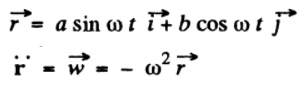15: Find the trajectory equation y (x) of a point if it moves according to the following laws: (a) x = a sin ωt, y = a sin 2ωt; (b) x = a sin cot, y = a cos 2ωt.

Solution:

1. (a) From the Equation: x = a sin ωt , we have cos ωt = √[1-(x2/a2)]

and from the Equation: y = a sin 2 ωt

y = 2a sin ωt cos ωt = 2x √[1-(x2/a2)] or y2 = 4x2 [1-(x2/a2)]

(b) From the Equation: x = a sin ωt

sin2 ωt = x2/a2

From y = a cos 2 ωt

y = a(1- 2sin2 ωt) = a √[1-(2x2/a2)]

16: A particle of mass m is located in a unidimensional potential field where the potential energy of the particle depends on the coordinate x as U (x) = U0 (1 - cos ax); U0 and a are constants. Find the period of small oscillations that the particle performs about the equilibrium position.

Solution:

1. As U (x) = U0 (1 - cos ax)

Fx = - dU/dx = -U0 a sin (ax)

For small angle of oscillations sin a x = a x

Fx = -U0 a2 x …..(1)

But we know, Fx = -mω02x , for small oscillation

Thus, ω02 = U0a2/m

Hence the sought time period is T = 2 π/ω0 = (2 π/a) √(m/Uo) = 2 π √(m/a2Uo)

17: Solve the foregoing problem if the potential energy has the form U (x) = alx2 - blx, where a and b are positive constants.

Solution:

1. U(x) = a/x2 - b/x

then the equilibrium position is x = x0 when U" (x0) = 0

or, -2a/x30 + b/x20 = 0

This implies, x0 = 2a/b

Now write: x = x0 + y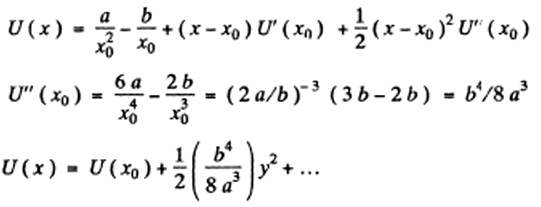Let us neglect remaining terms for small oscillations and compare with the P.E. for a harmonic, oscillator: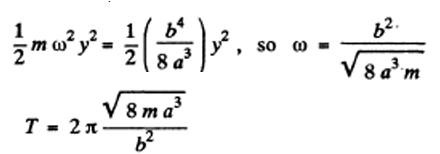* The answer given in the book is incorrect both numerically and dimensionally.

18: Find the period of small oscillations in a vertical plane performed by a ball of mass m = 40 g fixed at the middle of a horizontally stretched string l = 1.0 m in length. The tension of the string is assumed to be constant and equal to F = 10 N.

Solution:

1. Let us locate and depict the forces acting on the ball at the position when it is at a distance x down from the unreformed position of the string.

At this position, the unbalanced downward force on the ball = mg - 2Fsinθ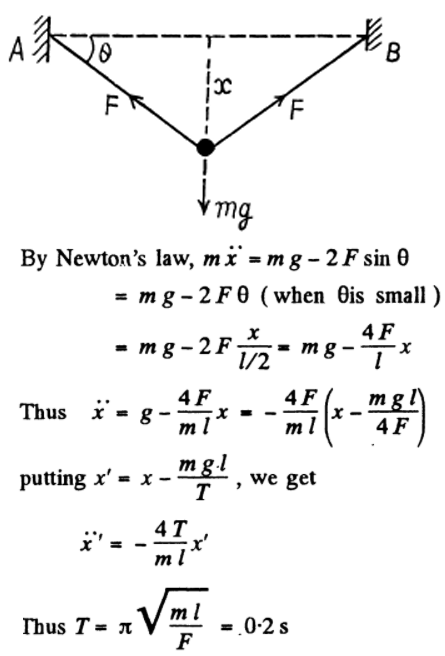19: Determine the period of small oscillations of a mathematical pendulum, that is a ball suspended by a thread l = 20 cm in length, if it is located in a liquid whose density is η = 3.0 times less than that of the ball. The resistance of the liquid is to be neglected.

Solution:

1. Let us depict the forces acting on the oscillating ball at an arbitrary angular position 0. (Figure below), relative to equilibrium position where FB is the force of buoyancy. For the ball from the equation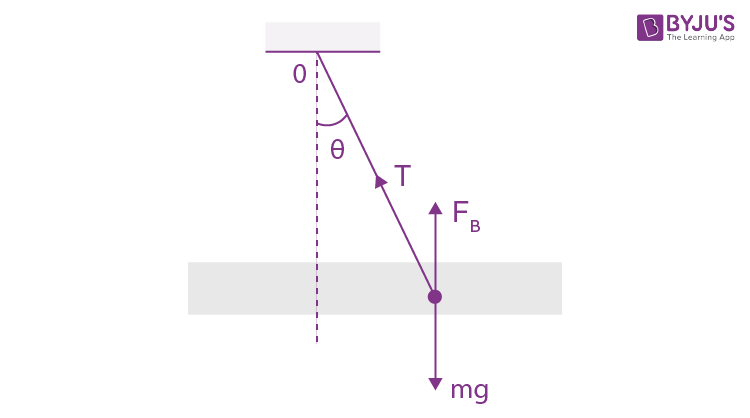Nz - Iβz (where we have taken the positive sense of Z axis in the direction of angular velocity of the ball and passes through the point of suspension of the pendulum O)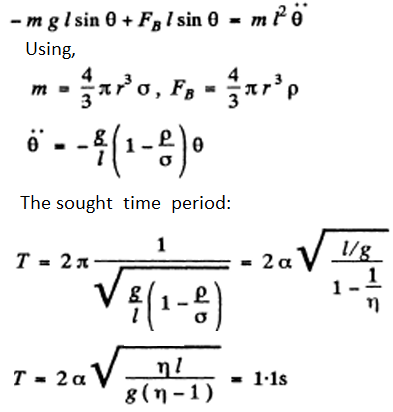(sin θ = θ, for small θ)

20: A ball is suspended by a thread of length l at the point 0 on the wall, forming a small angle α with the vertical (Fig. 4.1). Then the thread with the ball was deviated through a small angle β(β > α) and set free. Assuming the collision of the ball against the wall to be perfectly elastic, find the oscillation period of such a pendulum.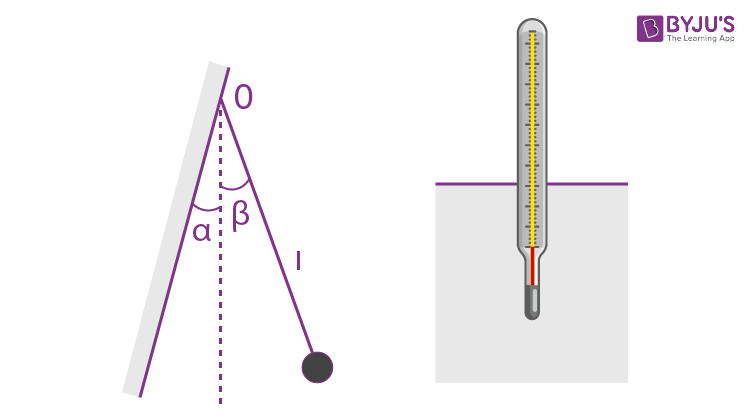Solution:

1. Obviously for small p the ball execute part of S.H.M. Due to the perfectly elastic collision the velocity of ball simply reversed. As the ball is in S.H.M. (|θ| < α on the left) its motion law in differential from can be written as

$$\ddot{\theta} = -\frac{g}{l} \theta = -\omega_0^2 \theta$$
.....(1)

If we assume that the ball is released from the extreme position, θ = β at t = 0, the solution of differential equation would be taken in the form

$$\theta = \beta cos \omega_0 \ t = \beta cos\sqrt{g/l}t$$
....(2)

If t' be the time taken by the ball to go from the extreme position θ = β to the wall i.e. θ = -α, then Equation (2) can be rewritten as